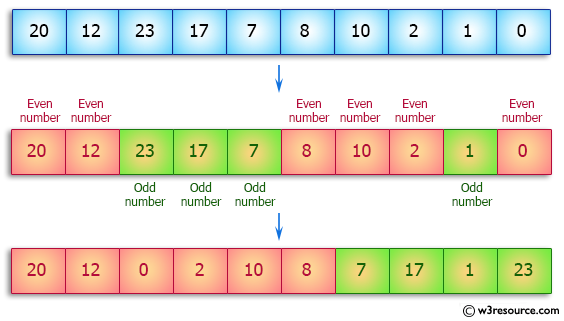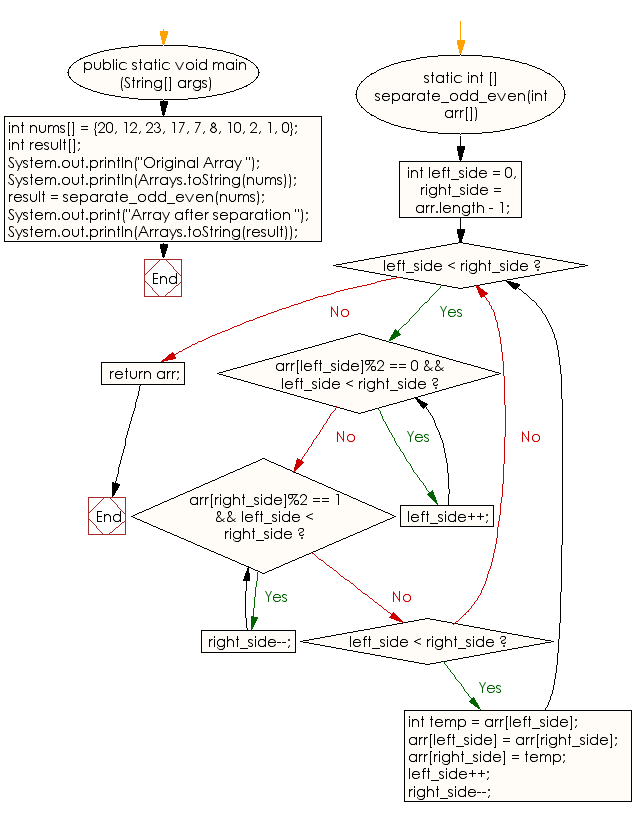﻿ Java: Separate even and odd numbers of a given array# Java Array Exercises: Separate even and odd numbers of a given array of integers

## Java Array: Exercise-52 with Solution

Write a Java program to separate even and odd numbers of a given array of integers. Put all even numbers first, and then odd numbers.

Pictorial Presentation:Sample Solution:

Java Code:

``````import java.util.Arrays;
public class Main
{

public static void main (String[] args)
{
int nums[] = {20, 12, 23, 17, 7, 8, 10, 2, 1, 0};
int result[];
System.out.println("Original Array ");
System.out.println(Arrays.toString(nums));

result = separate_odd_even(nums);

System.out.print("Array after separation ");
System.out.println(Arrays.toString(result));
}

static int [] separate_odd_even(int arr[])
{
int left_side = 0, right_side = arr.length - 1;
while (left_side < right_side)
{
while (arr[left_side]%2 == 0 && left_side < right_side)
left_side++;

while (arr[right_side]%2 == 1 && left_side < right_side)
right_side--;

if (left_side < right_side)
{
int temp = arr[left_side];
arr[left_side] = arr[right_side];
arr[right_side] = temp;
left_side++;
right_side--;
}
}
return arr;
}
}
```
```

Sample Output:

```
Original Array
[20, 12, 23, 17, 7, 8, 10, 2, 1, 0]
Array after separation [20, 12, 0, 2, 10, 8, 7, 17, 1, 23]
```

Flowchart:Visualize Java code execution (Python Tutor):

Java Code Editor:

Improve this sample solution and post your code through Disqus

What is the difficulty level of this exercise?

Test your Programming skills with w3resource's quiz.

﻿

## Java: Tips of the Day

countOccurrences

Counts the occurrences of a value in an array.

Use Arrays.stream().filter().count() to count total number of values that equals the specified value.

```public static long countOccurrences(int[] numbers, int value) {
return Arrays.stream(numbers)
.filter(number -> number == value)
.count();
}
```

Ref: https://bit.ly/3kCAgLb